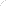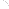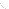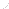Financial Data

[FEED NOT AVAILABLE]

FINANCIAL RATIOS

LIQUIDITY MEASURES

Current Ratio=Current Assets/Current Liabilities

Net Working Capital=Current Assets-Current Liabilities

ACTIVITY RATIOS

Accounts Receivable Turnover=Annual Sales/Accounts Receivables

Inventory Turnover=Annual Sales/Inventory

Total Asset Turnover=Annual Sales/Total Assets

LEVERAGE MEASURES

Debt-Equity Ratio=Long Term Debt/Shareholders Equity

Times Interest Earned=Earnings Before Interest and Taxes/Interest Expense

PROFITABILTY MEASURES

Net Profit Margin=Net Profit After Taxes/Total Revenue

Return on Assets(ROA)=Net Profit After Taxes/Total Assets

Return on Equity(ROE)=Net Profit After Taxes/Shareholders Equity

ROA=Net Profit Margin x Total Asset Turnover

ROE=ROA x Equity Multiplier

Equity Multiplier=Total Assets/Total Stockholders Equity

ROE=ROA x Equity Multiplier, Which =Net Profit Margin x Total Asset Turnover x Equity Multiplier

COMMON STOCK RATIOS

Price Earnings(PE)=Market Price of Stock/EPS

EPS=Net Profit After Taxes-Preferred Dividends/Number of Shares Outstanding

PEG Ratio=PE Ratio/3 to 5 Year Earnings Growth Rate

Dividends Per Share=Annual Dividends Paid to Common Stock/Number of Common Shares Outstanding

Payout Ratio=Dividends per Share/Earnings per Share

Book Value per Share=Common Stockholders Equity/Number of  Common Shares Outstanding

Price to Book Value=Market Price of Common Stock/Book Value per Share

Go To Top Of Page

Build your business with Email Marketing and Online Surveys from Constant Contact. FREE 60-Day Trial.Join Our Email List Email: